# Understanding Thermal Expansion with SOLIDWORKS Simulation

Article by John Nikoloff on May 04, 2022

I recently talked to a customer with validation concerns about an analysis - were the results and setup correct? With SOLIDWORKS Simulation and Simulation Professional, it is possible to verify the phenomenon of Thermal Expansion using Static Analysis tools. In this article, I'll compile the methods I used to diagnose and assist this customer's concern.

First, we will go over some theory and hand calculations, then, we will see how to properly set up our simulation and review the results.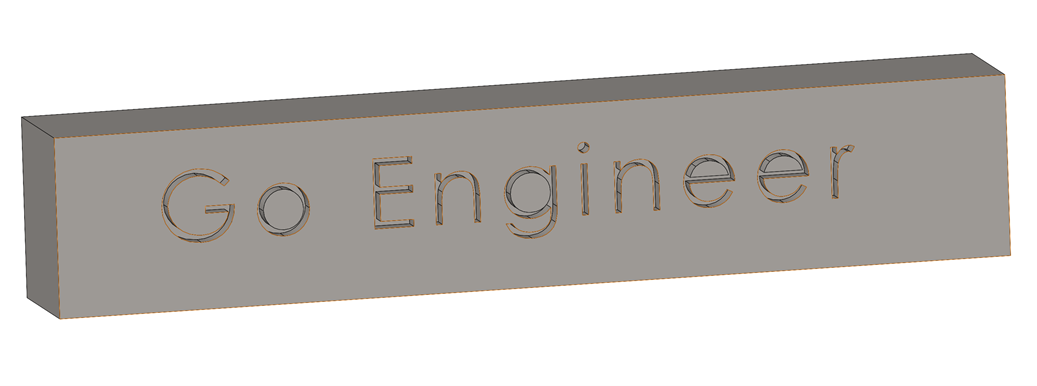## Theory and Hand Calculation

Let’s review the basic thermal expansion equation. If you recall, materials expand when introduced to thermal energy, causing the molecules to get excited and increase their total displacement within a lattice structure.

For linear expansion, we can illustrate thermal expansion as the fraction of change in length of a part being equal to the coefficient of expansion for that part’s material multiplied by the change in temperature of that part. Simply put, we illustrate this as: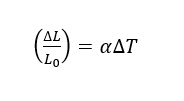(Figure 1.1)

For this example, we will introduce our part that is made of AISI 304 to thermal energy, raising its temerature to 100°C from 25°C. Where L0 is the length of our part, ΔL is the change in length that our part will undergo, ΔT is the change in temperature of our part, and α is our coefficient of thermal expansion. Given by our model, the initial length of our part, L0 = 50.88mm, α = 1.8 * 10 -5 K -1, and ΔT=75°C.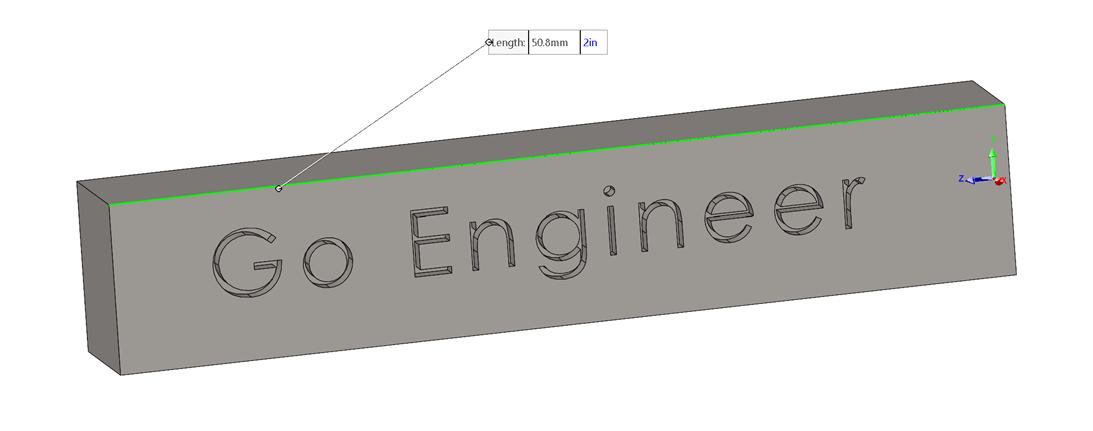(Figure 1.12)

To predict the resultant “displacement” of our part, we can simply rewrite this as: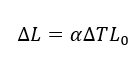(Figure 1.13)

Now, if we apply this equation to our given variables, we can write this as:

ΔL = (1.8 * 10 -5 K -1)(100°C - 25°C)(50.8mm) = 0.068 m

(Figure 1.14)

We will compare this resultant expanded length to our simulation next.

Once we have obtained our result from our Transient Thermal Stress analysis, let's confirm our result with a non-time dependent study.

Just like we did above, we will make a new Thermal study. Luckily, we do not need to change any of the study properties since a thermal analysis is static by default.

Going into the Thermal Loads data type, we can select the solid body that we will apply our 100°C load to.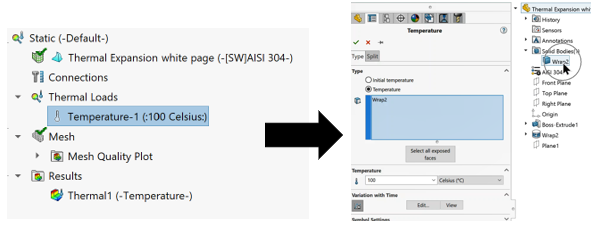(Figure 2)

We will run this study and load the thermal properties into a static stress analysis, just like before. Remember we are selecting the Solid Body of our model. This ensures we apply our thermal load to the entire model rather than the outside faces.

This method is also recommended for non-uniform temperatures being applied to the model.

## Thermal Static Analysis

We will follow the same procedure as we did for the Transient analysis > Static Stress analysis. We will also open the static stress analysis study properties and select Static to recall the thermal results of our non-time dependent thermal analysis (2.1). This setup is quite like before though we will neither select Transient nor a time step for our study.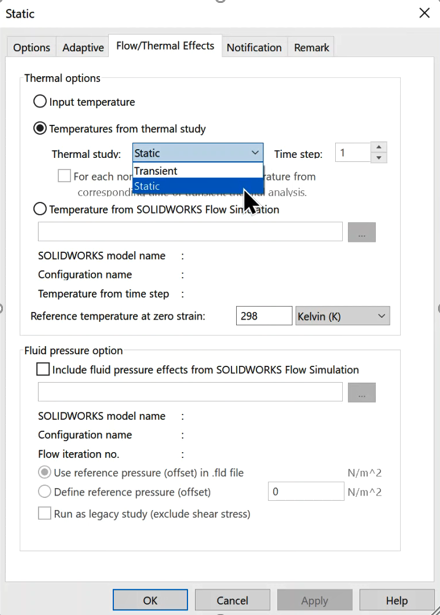(Figure 2.1)

After we set up the Static analysis (with the thermal loads of our Static Thermal Analysis), we can review the results.

First, I was able to get a total displacement (from the center of my model) of 0.069mm, only 0.001mm off with only a 1.47% error.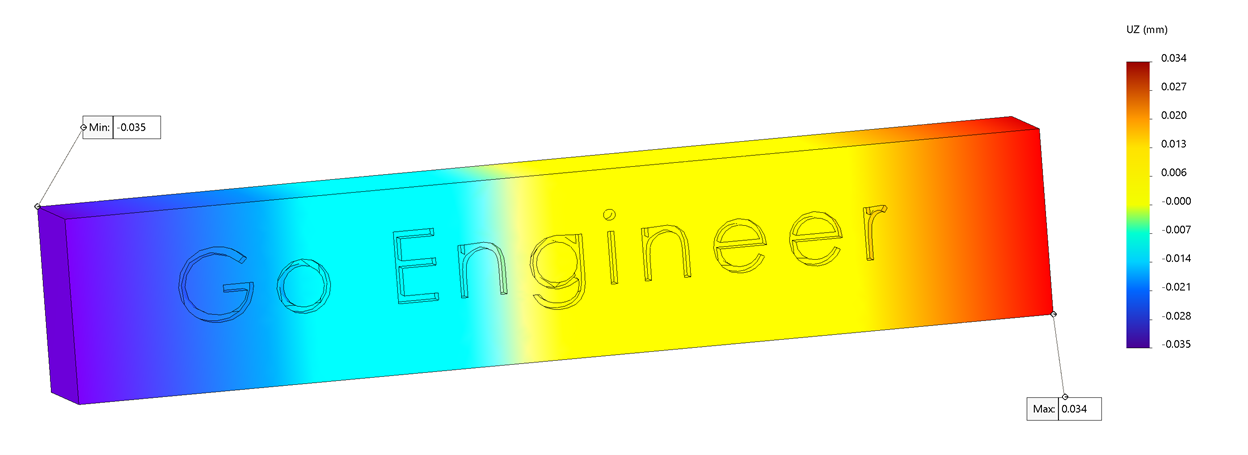## Conclusion

Luckily all I had to suggest to the customer was that they run the study as I did here. Although their model and results were different than mine, the method still applies. One could also simply run this analysis in a Thermal Static analysis. I hope this cleared up any confusion on how to run a thermal expansion analysis for your part! Check out more SOLIDWORKS Simulation articles below.

## More SOLIDWORKS Simulation Analysis Tutorials

7 Steps to Perform a Fatigue Analysis in SOLIDWORKS Simulation

Performing a Thermal Stress Analysis in SOLIDWORKS Simulation

How to Perform a Drop Test in SOLIDWORKS Simulation

Introduction to Structural Analysis

SOLIDWORKS Simulation Frequency Analysis Comparison Between FEA and Real Life